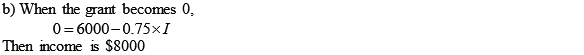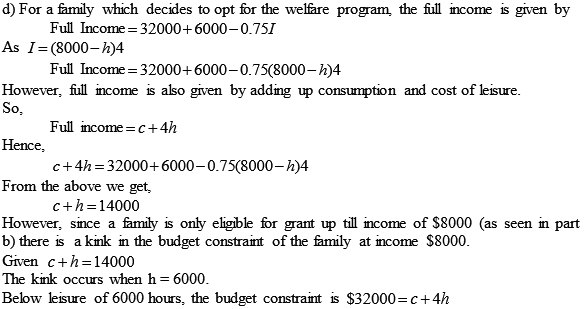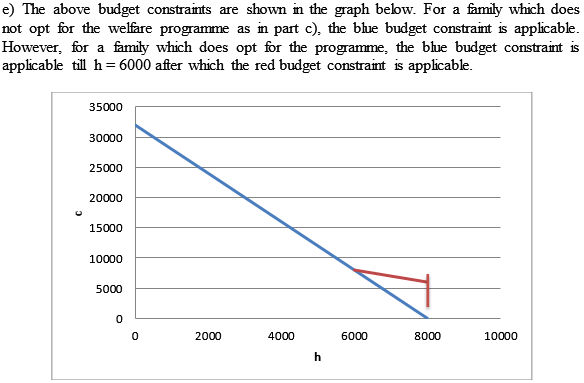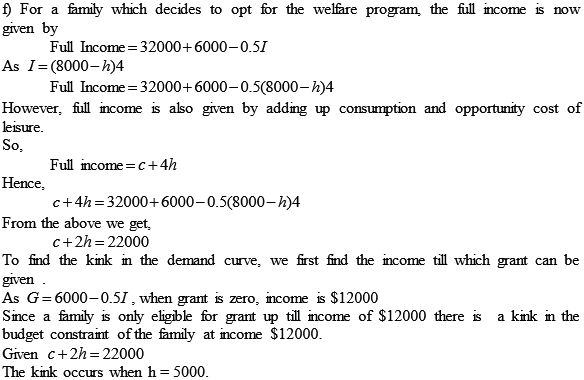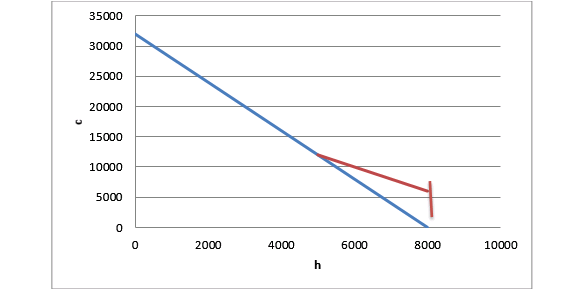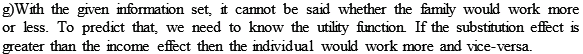## Quiz 15 : Labor MarketsLooking for Economics Homework Help?# Quiz 15 : Labor Markets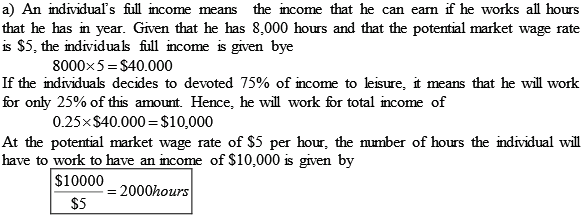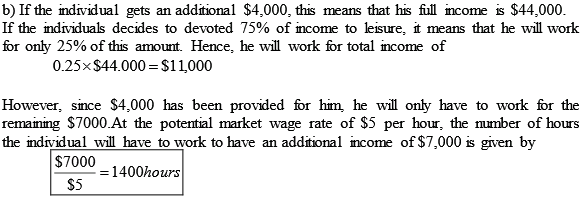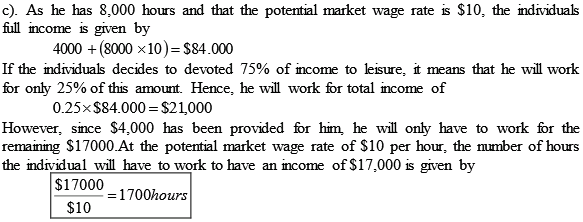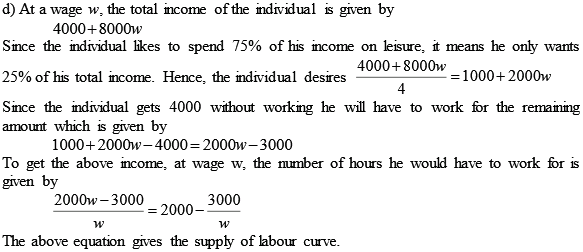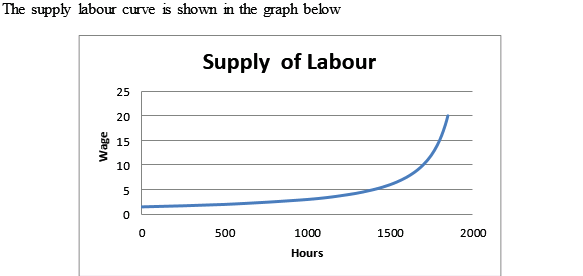In order to find the optimal consumption bundle an individual either can maximize his utility with given income or can minimize the expenditure to attain a particular level of utility. The utility function for an individual in terms of consumption and leisure is given below: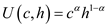The expenditure need to be minimized given the utility level at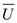. a. Setting the Lagrange equation to minimize the expenditure given utility level atas shown below: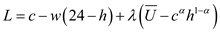The first order conditions of Lagrange equation can be computed as shown below:Set first order conditions equal to zero and solve it to get the value of optimal consumption bundle as shown below:Solving the first order differentials the value of c and h can be computed as shown below:The expenditure function can be shown as given below:b. The compensated demand function is the demand function for any commodity keeping the utility level at a particular constant level. The compensated demand function for leisure can be computed by using envelope theorem as shown below:The compensated demand function for consumption can't be computed as price of consumption here is treated as numeriare. c. The compensated labor supply function can be calculated as shown below:The derivative of compensated labor supply function with respect to w is shown below:Asand w is positive integer the value of compensated supply function will rise with rise in w that is the curve will be positively sloped. d. The compensated labor supply curve is given below: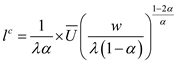The uncompensated labor supply is given below:The uncompensated demand curve is function of only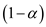that is the share of utility of leisure. Whereas the compensated demand curve is the function of constant utility and wage rate too. The Slutsky equation breaks the change in demand for one good in response to a change in the price of other good. The slustky equation is given below: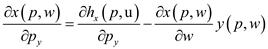In this case the income and substitution effect of real wages on uncompensated labor supply curve is offset by each other.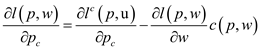Income effect of wage rise is zero.Substitution effect is also zero. So, rise in wages will not affect the uncompensated supply of labor.